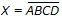# Digital Electronics - Logic Gates

### Exercise :: Logic Gates - General Questions

56.

CMOS IC packages are available in ________.

 A. DIP configuration B. SOIC configuration C. DIP and SOIC configurations D. neither DIP nor SOIC configurations

Explanation:

No answer description available for this question. Let us discuss.

57.

Which of the following is not a basic Boolean operation?

 A. OR B. NOT C. AND D. FOR

Explanation:

No answer description available for this question. Let us discuss.

58.

Which of the following gates is described by the expression?

 A. OR B. AND C. NOR D. NAND

Explanation:

No answer description available for this question. Let us discuss.

59.

What is the Boolean expression for a four-input OR gate?

 A. Y = A + B + C + D B. Y = A· B · C · D C. Y = A – B – C – D D. Y = A \$ B \$ C \$ D

Explanation:

No answer description available for this question. Let us discuss.

60.

How many truth table entries are necessary for a four-input circuit?

 A. 4 B. 8 C. 12 D. 16